Normal view MARC view ISBD view

# Introduction to functional data analysis

Material type:BookPublisher: Florida CRC Press 2017Description: xvi, 290p. With index.ISBN: 9781498746342.DDC classification: 515.7 Summary: Introduction to Functional Data Analysis provides a concise textbook introduction to the field. It explains how to analyze functional data, both at exploratory and inferential levels. It also provides a systematic and accessible exposition of the methodology and the required mathematical framework. The book can be used as textbook for a semester-long course on FDA for advanced undergraduate or MS statistics majors, as well as for MS and PhD students in other disciplines, including applied mathematics, environmental science, public health, medical research, geophysical sciences and economics. It can also be used for self-study and as a reference for researchers in those fields who wish to acquire solid understanding of FDA methodology and practical guidance for its implementation. Each chapter contains plentiful examples of relevant R code and theoretical and data analytic problems. The material of the book can be roughly divided into four parts of approximately equal length: 1) basic concepts and techniques of FDA, 2) functional regression models, 3) sparse and dependent functional data, and 4) introduction to the Hilbert space framework of FDA. The book assumes advanced undergraduate background in calculus, linear algebra, distributional probability theory, foundations of statistical inference, and some familiarity with R programming. Other required statistics background is provided in scalar settings before the related functional concepts are developed. Most chapters end with references to more advanced research for those who wish to gain a more in-depth understanding of a specific topic. https://www.crcpress.com/Introduction-to-Functional-Data-Analysis/Kokoszka-Reimherr/p/book/9781498746342
Tags from this library: No tags from this library for this title.
Item type Current location Item location Collection Call number Status Date due Barcode
Books Vikram Sarabhai Library
General Stacks
Slot 1365 (0 Floor, East Wing) Non-fiction 515.7 K6I6 (Browse shelf) Available 199254
##### Browsing Vikram Sarabhai Library Shelves , Shelving location: General Stacks , Collection code: Non-fiction Close shelf browser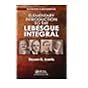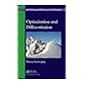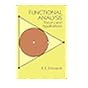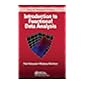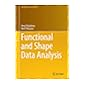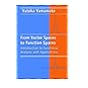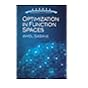515.43 K7E5 Elementary introduction to the Lebesgue integral 515.642 S3O7 Optimization and differentiation 515.7 E2F8 Functional analysis: theory and applications 515.7 K6I6 Introduction to functional data analysis 515.7 S7F8 Functional and shape data analysis 515.7 Y2F7 From vector spaces to function spaces: introduction to functional analysis with applications 515.73 S2O7 Optimization in function spaces

1 First steps in the analysis of functional data

2 Further topics in exploratory FDA

3 Mathematical framework for functional data

4 Scalar- on - function regression

5 Functional response models

6 Functional generalized linear models

7 Sparse FDA

8 Functional time series

9 Spatial functional data and models

10 Elements of Hilbert space theory

11 Random functions

12 Inference from a random sample

Introduction to Functional Data Analysis provides a concise textbook introduction to the field. It explains how to analyze functional data, both at exploratory and inferential levels. It also provides a systematic and accessible exposition of the methodology and the required mathematical framework. The book can be used as textbook for a semester-long course on FDA for advanced undergraduate or MS statistics majors, as well as for MS and PhD students in other disciplines, including applied mathematics, environmental science, public health, medical research, geophysical sciences and economics. It can also be used for self-study and as a reference for researchers in those fields who wish to acquire solid understanding of FDA methodology and practical guidance for its implementation. Each chapter contains plentiful examples of relevant R code and theoretical and data analytic problems. The material of the book can be roughly divided into four parts of approximately equal length: 1) basic concepts and techniques of FDA, 2) functional regression models, 3) sparse and dependent functional data, and 4) introduction to the Hilbert space framework of FDA. The book assumes advanced undergraduate background in calculus, linear algebra, distributional probability theory, foundations of statistical inference, and some familiarity with R programming. Other required statistics background is provided in scalar settings before the related functional concepts are developed. Most chapters end with references to more advanced research for those who wish to gain a more in-depth understanding of a specific topic.

https://www.crcpress.com/Introduction-to-Functional-Data-Analysis/Kokoszka-Reimherr/p/book/9781498746342

There are no comments for this item.